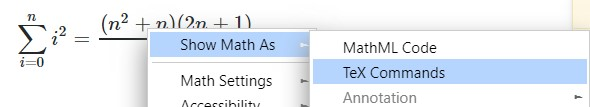# Example writing MathJax formulas

This would be MathJax basic tutorial and reference you may need frequently dealing with formulas.

## Use of $ and $$ You enclose inline formulas just with  (dollar sign): inline$$center$$$inline$$center$ ## Superscripts and Subscripts For superscripts and subscripts, use ^ and _. For example, x_i^2 would produce$x_i^2$. Wrap inside {} if you plan to have a complicated subscript with the superscript or vice versa. ## Controlling the sizes • \tiny{ a } =>$\tiny{ a }$• \scriptsize{ a }=>$\scriptsize{ a }$• \small{ a } =>$\small{a}$• \normalsize{ a } =>$\normalsize{a}$• \large{ a } =>$\large{a}$• \Large{ a } =>$\Large{a}$• \LARGE{ a } =>$\LARGE{a}$• \huge{ a } =>$\huge{a}$• \Huge{ a } =>$\Huge{a}$## Curly braces For curly braces${}$use \{ and \}. Curly braces are also used as teh grouping character. ## Fractions Couple ways to create fractions: • \frac ab =>$\frac ab$It works just for the two letters, so very inconvenient. You can use grouping using the curly braces: • \frac{a+1}{b+1} =>$\frac{a+1}{b+1}$Another way is to use \over: • {a+1\over b+1} =>${a+1\over b+1}$You can also use \cfrac: • \cfrac{a}{b} =>$\cfrac{a}{b}$Example: x = a_0 + \cfrac{1^2}{a_1 + \cfrac{2^2}{a_2 + \cfrac{3^2}{a_3 + \cfrac{4^4}{a_4 + \cdots}}}} $x = a_0 + \cfrac{1^2}{a_1 + \cfrac{2^2}{a_2 + \cfrac{3^2}{a_3 + \cfrac{4^4}{a_4 + \cdots}}}}$## Appendix ### Getting the formula from other website: Right click the formula and select Show Math As > TeX Commands.### Greek letters Use: • \alpha =>$\alpha$• \beta =>$\beta$• \gama =>$\gamma$• \delta =>$\delta$• \omega =>$\omega$for lowercase and • \Gamma =>$\Gamma$• \Delta =>$\Delta$• \Omega =>$\Omega$for uppercase greek letters. ### Injecting MathJax on webpage <script type="text/x-mathjax-config"> MathJax.Hub.Config({ tex2jax: { skipTags: ['script', 'noscript', 'style', 'textarea', 'pre'], inlineMath: [['$','\$']]